## 5.66. circuit

Origin
Constraint

$\mathrm{𝚌𝚒𝚛𝚌𝚞𝚒𝚝}\left(\mathrm{𝙽𝙾𝙳𝙴𝚂}\right)$

Synonyms

$\mathrm{𝚊𝚝𝚘𝚞𝚛}$, $\mathrm{𝚌𝚢𝚌𝚕𝚎}$.

Argument
 $\mathrm{𝙽𝙾𝙳𝙴𝚂}$ $\mathrm{𝚌𝚘𝚕𝚕𝚎𝚌𝚝𝚒𝚘𝚗}\left(\mathrm{𝚒𝚗𝚍𝚎𝚡}-\mathrm{𝚒𝚗𝚝},\mathrm{𝚜𝚞𝚌𝚌}-\mathrm{𝚍𝚟𝚊𝚛}\right)$
Restrictions
 $\mathrm{𝚛𝚎𝚚𝚞𝚒𝚛𝚎𝚍}$$\left(\mathrm{𝙽𝙾𝙳𝙴𝚂},\left[\mathrm{𝚒𝚗𝚍𝚎𝚡},\mathrm{𝚜𝚞𝚌𝚌}\right]\right)$ $\mathrm{𝙽𝙾𝙳𝙴𝚂}.\mathrm{𝚒𝚗𝚍𝚎𝚡}\ge 1$ $\mathrm{𝙽𝙾𝙳𝙴𝚂}.\mathrm{𝚒𝚗𝚍𝚎𝚡}\le |\mathrm{𝙽𝙾𝙳𝙴𝚂}|$ $\mathrm{𝚍𝚒𝚜𝚝𝚒𝚗𝚌𝚝}$$\left(\mathrm{𝙽𝙾𝙳𝙴𝚂},\mathrm{𝚒𝚗𝚍𝚎𝚡}\right)$ $\mathrm{𝙽𝙾𝙳𝙴𝚂}.\mathrm{𝚜𝚞𝚌𝚌}\ge 1$ $\mathrm{𝙽𝙾𝙳𝙴𝚂}.\mathrm{𝚜𝚞𝚌𝚌}\le |\mathrm{𝙽𝙾𝙳𝙴𝚂}|$
Purpose

Enforce to cover a digraph $G$ described by the $\mathrm{𝙽𝙾𝙳𝙴𝚂}$ collection with one circuit visiting once all vertices of $G$.

Example
$\left(\begin{array}{c}〈\begin{array}{cc}\mathrm{𝚒𝚗𝚍𝚎𝚡}-1\hfill & \mathrm{𝚜𝚞𝚌𝚌}-2,\hfill \\ \mathrm{𝚒𝚗𝚍𝚎𝚡}-2\hfill & \mathrm{𝚜𝚞𝚌𝚌}-3,\hfill \\ \mathrm{𝚒𝚗𝚍𝚎𝚡}-3\hfill & \mathrm{𝚜𝚞𝚌𝚌}-4,\hfill \\ \mathrm{𝚒𝚗𝚍𝚎𝚡}-4\hfill & \mathrm{𝚜𝚞𝚌𝚌}-1\hfill \end{array}〉\hfill \end{array}\right)$

The $\mathrm{𝚌𝚒𝚛𝚌𝚞𝚒𝚝}$ constraint holds since its $\mathrm{𝙽𝙾𝙳𝙴𝚂}$ argument depicts the following Hamiltonian circuit visiting successively the vertices 1, 2, 3, 4 and 1.

All solutions

Figure 5.66.1 gives all solutions to the following non ground instance of the $\mathrm{𝚌𝚒𝚛𝚌𝚞𝚒𝚝}$ constraint: ${S}_{1}\in \left[3,4\right]$, ${S}_{2}\in \left[1,2\right]$, ${S}_{3}\in \left[1,4\right]$, ${S}_{4}\in \left[2,4\right]$, $\mathrm{𝚌𝚒𝚛𝚌𝚞𝚒𝚝}$$\left(〈1{S}_{1},2{S}_{2},3{S}_{3},4{S}_{4}〉\right)$.

##### Figure 5.66.1. All solutions corresponding to the non ground example of the $\mathrm{𝚌𝚒𝚛𝚌𝚞𝚒𝚝}$ constraint of the All solutions slot (the $\mathrm{𝚒𝚗𝚍𝚎𝚡}$ attribute is displayed as indices of the $\mathrm{𝚜𝚞𝚌𝚌}$ attribute)Typical
$|\mathrm{𝙽𝙾𝙳𝙴𝚂}|>2$
Symmetry

Items of $\mathrm{𝙽𝙾𝙳𝙴𝚂}$ are permutable.

Remark

In the original $\mathrm{𝚌𝚒𝚛𝚌𝚞𝚒𝚝}$ constraint of CHIP the $\mathrm{𝚒𝚗𝚍𝚎𝚡}$ attribute was not explicitly present. It was implicitly defined as the position of a variable in a list.

Within the context of linear programming [AlthausBockmayrElfKasperJungerMehlhorn02] this constraint was introduced under the name $\mathrm{𝚊𝚝𝚘𝚞𝚛}$. In the same context [Hooker07book] provides continuous relaxations of the $\mathrm{𝚌𝚒𝚛𝚌𝚞𝚒𝚝}$ constraint.

Within the KOALOG constraint system this constraint is called $\mathrm{𝚌𝚢𝚌𝚕𝚎}$.

Algorithm

Since all $\mathrm{𝚜𝚞𝚌𝚌}$ variables of the $\mathrm{𝙽𝙾𝙳𝙴𝚂}$ collection have to take distinct values one can reuse the algorithms associated with the $\mathrm{𝚊𝚕𝚕𝚍𝚒𝚏𝚏𝚎𝚛𝚎𝚗𝚝}$ constraint. A second necessary condition is to have no more than one strongly connected component. Pruning for enforcing this condition can be done by forcing all strong bridges to belong to the final solution, since otherwise the strongly connected component would be broken apart. A third necessary condition is that, if the graph is bipartite then the number of vertices of each class should be identical. Consequently if the number of vertices is odd (i.e., $|\mathrm{𝙽𝙾𝙳𝙴𝚂}|$ is odd) the graph should not be bipartite. Further necessary conditions (useful when the graph is sparse) combining the fact that we have a perfect matching and a single strongly connected component can be found in [ShufetBerliner94]. These conditions forget about the orientation of the arcs of the graph and characterise new required elementary chains. A typical pattern involving four vertices is depicted by Figure 5.66.2 where we assume that:

• There is an elementary chain between $c$ and $d$ (depicted by a dashed edge),

• $b$ has exactly 3 neighbours.

In this context the edge between $a$ and $b$ is mandatory in any covering (i.e., the arc from $a$ to $b$ or the arc from $b$ to $a$) since otherwise a small circuit involving $b$, $c$ and $d$ would be created.

##### Figure 5.66.2. Reasoning about elementary chains and degrees: if we have an elementary chain between $c$ and $d$ and if $b$ has 3 neighbours then the edge $\left(a,b\right)$ is mandatory.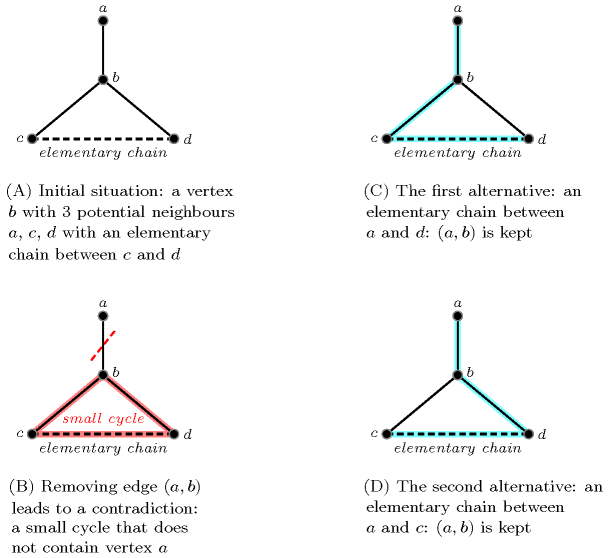When the graph is planar [HopcroftTarjan74][Deo76] one can also use as a necessary condition discovered by Grinberg [Grinberg68] for pruning.

Finally, another approach based an the notion of 1-toughness [Chvatal73] was proposed in [KayaHooker06] and evaluated for small graphs (i.e., graphs with up to 15 vertices).

Reformulation

Let $n$ and ${s}_{1},{s}_{2},\cdots ,{s}_{n}$ respectively denotes the number of vertices (i.e., $|\mathrm{𝙽𝙾𝙳𝙴𝚂}|$) and the successor variables associated with vertices $1,2,\cdots ,n$. The $\mathrm{𝚌𝚒𝚛𝚌𝚞𝚒𝚝}$ constraint can be reformulated as a conjunction of one $\mathrm{𝚍𝚘𝚖𝚊𝚒𝚗}$ constraint, two $\mathrm{𝚊𝚕𝚕𝚍𝚒𝚏𝚏𝚎𝚛𝚎𝚗𝚝}$ constraints, and $n$ $\mathrm{𝚎𝚕𝚎𝚖𝚎𝚗𝚝}$ constraints.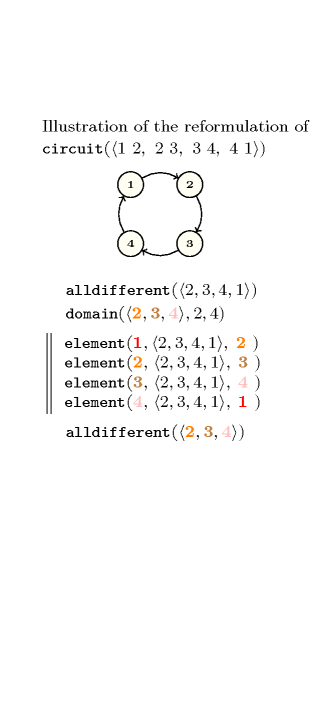Counting
 Length ($n$) 2 3 4 5 6 7 8 9 10 Solutions 1 2 6 24 120 720 5040 40320 362880

Number of solutions for $\mathrm{𝚌𝚒𝚛𝚌𝚞𝚒𝚝}$: domains $0..n$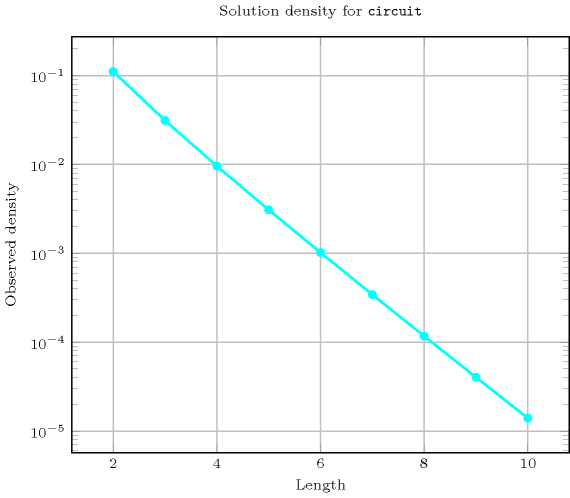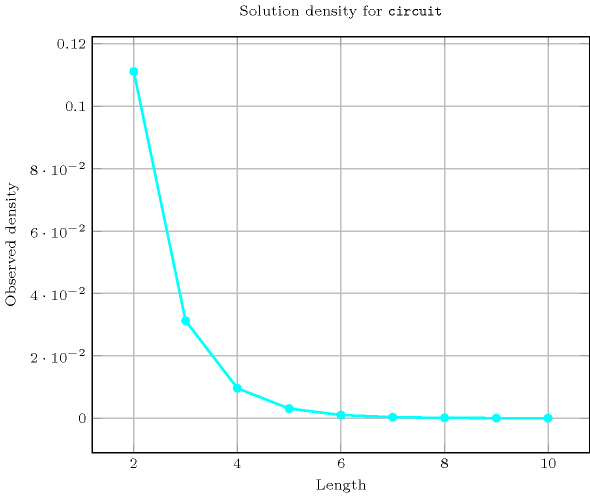Systems

generalisation: $\mathrm{𝚌𝚢𝚌𝚕𝚎}$ (introduce a variable for the number of circuits).

Keywords
Cond. implications

$•$ $\mathrm{𝚌𝚒𝚛𝚌𝚞𝚒𝚝}\left(\mathrm{𝙽𝙾𝙳𝙴𝚂}\right)$

implies $\mathrm{𝚌𝚢𝚌𝚕𝚎}$$\left(\mathrm{𝙽𝙲𝚈𝙲𝙻𝙴},\mathrm{𝙽𝙾𝙳𝙴𝚂}\right)$

when  $\mathrm{𝙽𝙲𝚈𝙲𝙻𝙴}=1$.

$•$ $\mathrm{𝚌𝚒𝚛𝚌𝚞𝚒𝚝}\left(\mathrm{𝙽𝙾𝙳𝙴𝚂}\right)$

with  $|\mathrm{𝙽𝙾𝙳𝙴𝚂}|>1$

implies $\mathrm{𝚍𝚎𝚛𝚊𝚗𝚐𝚎𝚖𝚎𝚗𝚝}$$\left(\mathrm{𝙽𝙾𝙳𝙴𝚂}\right)$.

$•$ $\mathrm{𝚌𝚒𝚛𝚌𝚞𝚒𝚝}\left(\mathrm{𝙽𝙾𝙳𝙴𝚂}\right)$

with  $|\mathrm{𝙽𝙾𝙳𝙴𝚂}|>1$

implies $𝚔_\mathrm{𝚊𝚕𝚕𝚍𝚒𝚏𝚏𝚎𝚛𝚎𝚗𝚝}$$\left(\mathrm{𝚅𝙰𝚁𝚂}:\mathrm{𝙽𝙾𝙳𝙴𝚂}\right)$.

$•$ $\mathrm{𝚌𝚒𝚛𝚌𝚞𝚒𝚝}\left(\mathrm{𝙽𝙾𝙳𝙴𝚂}\right)$

implies $\mathrm{𝚙𝚎𝚛𝚖𝚞𝚝𝚊𝚝𝚒𝚘𝚗}$$\left(\mathrm{𝚅𝙰𝚁𝙸𝙰𝙱𝙻𝙴𝚂}:\mathrm{𝙽𝙾𝙳𝙴𝚂}\right)$.

Arc input(s)

$\mathrm{𝙽𝙾𝙳𝙴𝚂}$

Arc generator
$\mathrm{𝐶𝐿𝐼𝑄𝑈𝐸}$$↦\mathrm{𝚌𝚘𝚕𝚕𝚎𝚌𝚝𝚒𝚘𝚗}\left(\mathrm{𝚗𝚘𝚍𝚎𝚜}\mathtt{1},\mathrm{𝚗𝚘𝚍𝚎𝚜}\mathtt{2}\right)$

Arc arity
Arc constraint(s)
$\mathrm{𝚗𝚘𝚍𝚎𝚜}\mathtt{1}.\mathrm{𝚜𝚞𝚌𝚌}=\mathrm{𝚗𝚘𝚍𝚎𝚜}\mathtt{2}.\mathrm{𝚒𝚗𝚍𝚎𝚡}$
Graph property(ies)
 $•$$\mathrm{𝐌𝐈𝐍}_\mathrm{𝐍𝐒𝐂𝐂}$$=|\mathrm{𝙽𝙾𝙳𝙴𝚂}|$ $•$$\mathrm{𝐌𝐀𝐗}_\mathrm{𝐈𝐃}$$\le 1$

Graph class
$\mathrm{𝙾𝙽𝙴}_\mathrm{𝚂𝚄𝙲𝙲}$

Graph model

The first graph property enforces to have a single strongly connected component containing $|\mathrm{𝙽𝙾𝙳𝙴𝚂}|$ vertices. The second graph property imposes to only have circuits. Since each vertex of the final graph has only one successor we do not need to use set variables for representing the successors of a vertex.

Parts (A) and (B) of Figure 5.66.3 respectively show the initial and final graph associated with the Example slot. The $\mathrm{𝚌𝚒𝚛𝚌𝚞𝚒𝚝}$ constraint holds since the final graph consists of one circuit mentioning once every vertex of the initial graph.

##### Figure 5.66.3. Initial and final graph of the $\mathrm{𝚌𝚒𝚛𝚌𝚞𝚒𝚝}$ constraint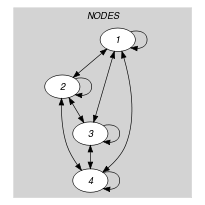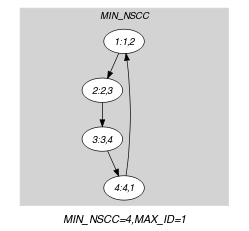(a) (b)